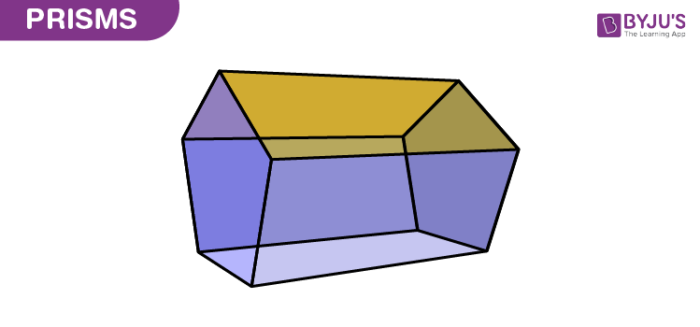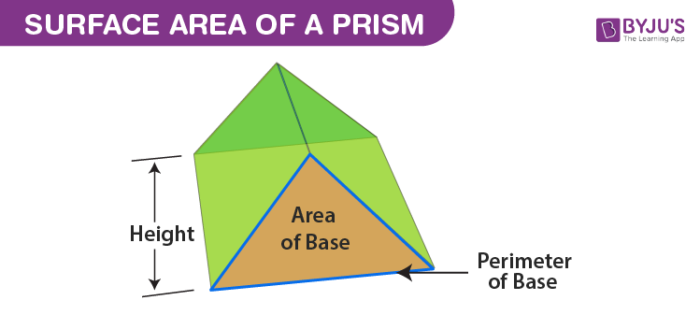# MTH2: VOLUME

##### This unit is about Volume of different objects and their surface areas.

Prism is a three-dimensional solid object in which the two ends are exactly of the same shape. It is the combination of the flat faces, identical bases and same cross-sections. The faces are parallelograms without the bases. If you take the cross-section of the prism parallel to the bases, the cross-sections will look like the bases. In this article, let us have a complete explanation about the types and also how to find the volume and area of a prism.

## Prism Shape

A prism has a solid shape consisting of two equal ends, flat faces or surfaces and identical cross-section across its length. The cross-section looks like a triangle hence called triangular prism. The shape of the prism does not have any curve. Hence, a prism can have cubic, rectangular, pentagonal and other polygon shapes but not the circular shape.## Types of Prism

Depending upon the cross-sections, the prisms are named. It is of two types, namely;

• Regular Prism
• Irregular Prism

### Regular Prism

If the bases of the prism are in the shape of a regular polygon, it is called regular prism.

### Irregular Prism

If the bases are in the shape of an irregular polygon, then the prism is called an irregular prism.

### Right Prism And Oblique Prism

Apart from regular and irregular, the prism can be classified into two more types;

• Right Prism
• Oblique Prism

The difference between both the prism are;

 Right Prism Oblique Prism If the faces and the joining edges are perpendicular to the base faces, then it is known as right prism If the faces and the joining edges are not perpendicular to the base faces, then it is known as oblique prism In right prism, the side faces are rectangles In an oblique prism, the side faces are parallelograms

Based on the shape of the bases, it is further categorised into different types, namely;

• Triangular
• Square
• Rectangular
• Pentagonal
• Hexagonal

## Prism Formulas

The formulas are defined for the surface area and volume of the prism. As the prism is a three-dimensional shape, so it has both the properties, i.e., surface area and volume.

### Surface Area of a Prism

The surface area of the prism is the total area covered by the faces of the prism.For any kind of prism, the surface area can be found using the formula;

 Surface Area of a Prism = 2(Base Area)+ (Base perimeter × length)

### Volume of a Prism

The volume of the prism is defined as the product of the base area and the prism height

Therefore,

 Volume of Prism = Base Area × Height

For example, if you want to find the volume of a square prism, you must know the area of a square, then its volume can be calculated as follows:

The volume of a square Prism = Area of square×height

V = s2 × h cubic units

Where “s” is the side of a square.

### Prism Problems

Example 1: Find the volume of a triangular prism whose area is 60cm2 and height is 7cm?

Solution: Given,

Area = 60 cm2

Height = 7 cm

We know that,

The volume of a prism = Base area × Height cubic units

Therefore, V = 60 ×7 = 420

Hence, the volume of a triangular prism = 420 cm3.

Example 2: Find the height of the square prism whose volume is 360 cm3 and the base area is 60 cm2?

Solution: Given,

The volume of a square prism = 360cm3

Base Area = 60cm2

Therefore, the height of the square prism is calculated as follows:

The volume of square prism = Base area × height

360 = 60 × prism height

Therefore, the height,h = 360/60

Prism Height, h = 6 cm.

A VIDEO ABOUT VOLUME OF DIFFERENT OBJECTS

### ASSIGNMENT : VOLUME ASSIGNMENTMARKS : 30  DURATION : 2 hours

SEE ALL
•YOU